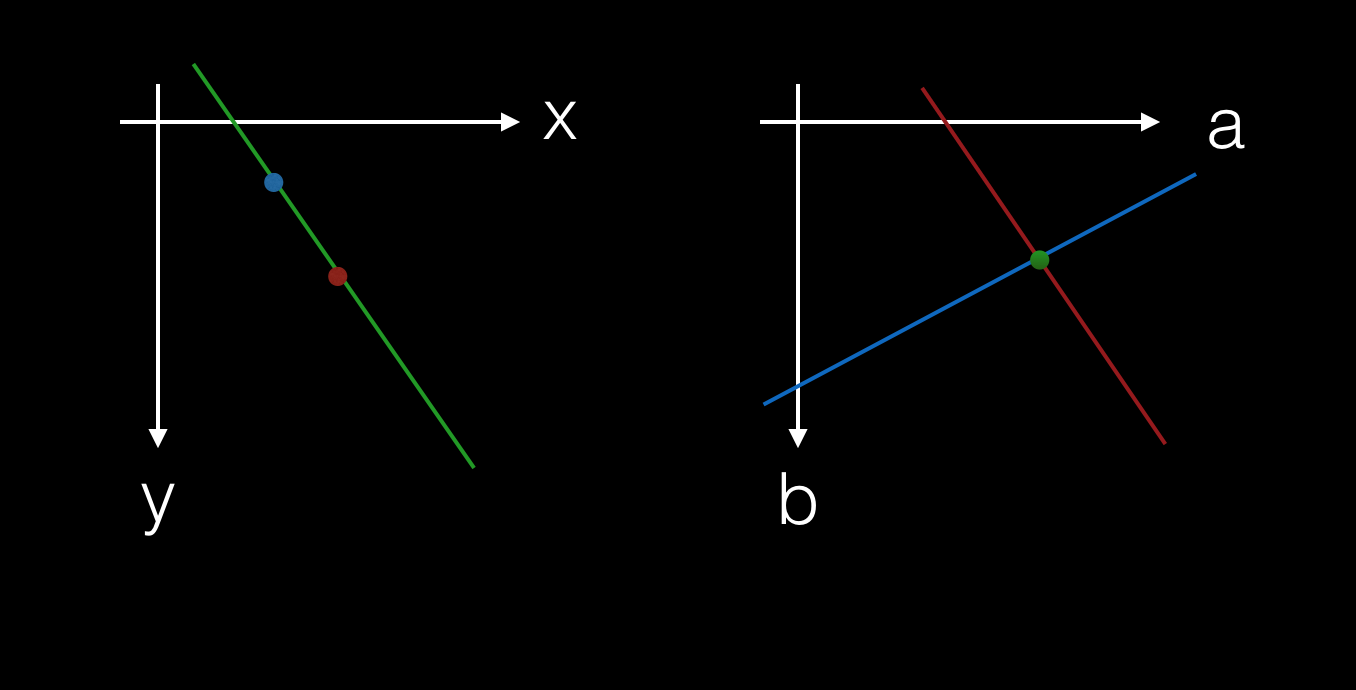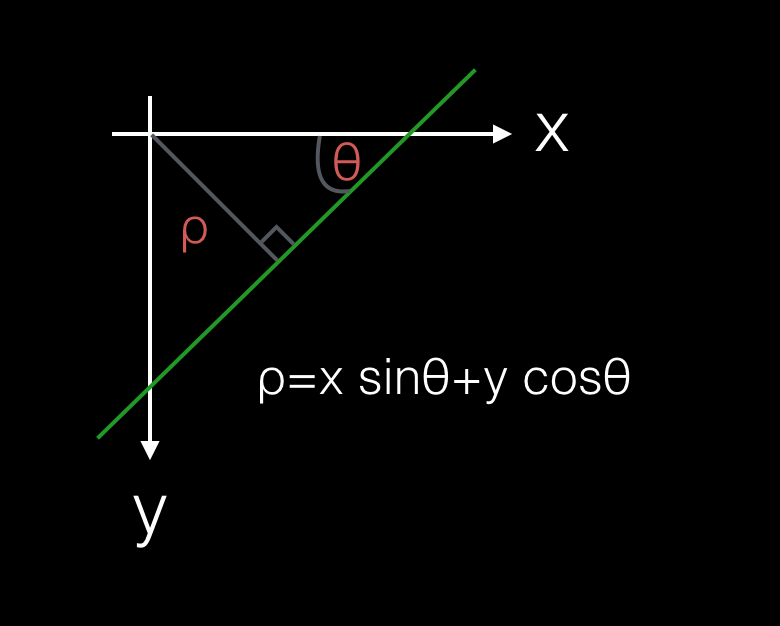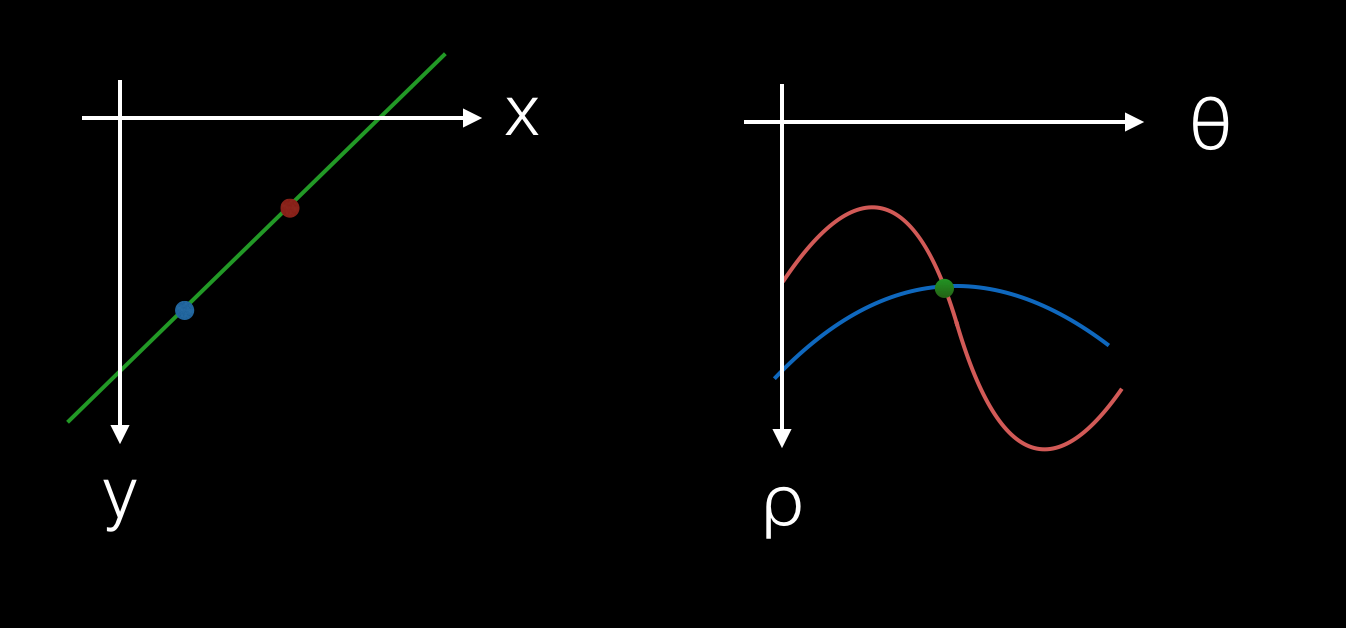# The Hough Transform

Recently, I wanted to use my laptop camera to detect a chess-board. One of the image processing techniques I found was the Hough Transform*, which is a way of detecting simple shapes, in my case lines, in an image. Surprisingly, it’s not all that hard to understand and I’ll try here to explain it.

Here’s an image of a book that I took with my laptop camera.

[imagine a picture here]

First we’re going to convert it to grayscale, blur it a little and run an edge detection algorithm over it. I won’t cover these steps here, but this is what it looks like afterwards:

[imagine a picture here]

We can ignore the black pixels, and treat the white pixels as a set of $(x,y)$ coordinates. We’d like to find a set of lines in this image, such that each line goes through a minimum number of white pixels that we’ll choose - let’s say 20 pixels for now.

Each individual point sits on an infinity of lines. So, unfortunately, we can’t check all the lines a point sits on and compare them to all the lines another point sits on. How can we reduce the scope of this task? This is where the Hough Transform comes in.

First of all, consider that a straight line can be represented as an equation in the form $y = ax + b$. Two points on the same line can be seen as two possible solutions to an equation of that form with a particular a and b.

We’re used to seeing x-y plots, but let’s consider what we’d see if we plotted a-b from that line equation instead.

We’ve already said that a point sits on an infinity of lines and each line is represented by a particular a and b value. It’s not too hard to see that a point in x-y becomes a straight line in the a-b parameter space.Let’s just check that, using the power of maths…

Say we take the point $(-2,4)$. Substituting into $y = ax + b$. We get $4 = -2a + b$. And with a bit of rearranging, I hope you can see that we end up with $a = b/2 - 2$, the equation of a straight line in a-b. And this works for every point.

Further, where two lines cross in a-b, their intersection (or intercept) represents a line in x-y that both x-y points are on. In some sense, when we go from x-y to a-b, points become lines and lines become points.

So, all we need to do is tranform points into the a-b parameter space and find the places where 5 or more a-b lines cross. Those intersections will have a-b values that give us the lines in x-y space with 5 or more pixels on them.

This is the essence of the Hough transform - we tranform points into a parameter-space where they become lines and find and rank the intersections.

But hold on a second, while this gives us an intuition for the Hough transform, it’s hard to do in practice. Since the slope of a line can go to infinity, searching the a-b space for lines that cross isn’t really possible.

Thankfully, there’s another way to represent lines and another parameter space we can use that’s less problematic. Lines in x-y can also be represented by the equation: $\rho = x sin\theta + y cos\theta$ where $\rho$ is the distance from the origin to the closest point on the line and $\theta$ is the angle between the x-axis and that line.And we can do the same ‘x-y to a-b’ trick that we did above. In the $\theta-\rho$ parameter-space, points also become lines, but this time they’re not straight, but rather each point traces a sinusoidal curve.By quantizing (ie. rounding) these parameters, we can create a bounded set of $(\theta,\rho)$ pairs for each point in the image, and those pairs that appear in 5 or more sets are our lines. This is much easier to search for intersections!

In practice, what we’ll do is build a set of $(\theta,\rho)$ pairs and accumulate into it over all the points, such that end up with a set of pairs and a count of the number of times each different point’s curves go through that pair.

Let’s look at some example code that might do this:

function houghTransform(pixels, threshold) {
var hough = {};

pixels.forEach(function(pixel) {
for (var theta = 0; theta < 2 * Math.PI; theta += 0.05) {
// Math.round is how we 'quantize' rho values
var rho = Math.round(pixel.x * Math.cos(theta) + pixel.y * Math.sin(theta));
if (rho > 0) {
var key = theta.toString() + "," + rho.toString();
if (hough[key] == undefined) {
hough[key] = { theta: theta, rho: rho, count: 1 }
} else {
hough[key].count += 1
}
}
}
})

return Object.values(hough).filter(function(tr) {
return (tr.count > threshold)
})
}


[imagine a demo using webcam here]

* I understand that ‘Hough’ is pronounced like ‘tough’. Which is a shame. If it were pronounced like ‘plough’, then we could make the alaska/jamaica joke:
“How can I detect simple lines in an image?”
“I don’t know, Hough?”
“Well, that’s what I’m asking! Also, what is the SI unit of power?”
Admittedly, computer vision experts probably have better jokes than this.

Written on November 12, 2016